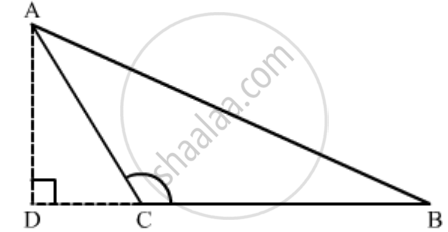Advertisement Remove all ads

# In ∆Abc, ∠C is an Obtuse Angle. Ad ⊥ Bc and Ab2 = Ac2 + 3 Bc2. Prove that Bc = Cd. - Mathematics

Sum

In ∆ABC, ∠C is an obtuse angle. AD ⊥ BC and AB2 = AC2 + 3 BC2. Prove that BC = CD.

Advertisement Remove all ads

#### Solution

GivenΔABC where C is an obtuse angle, AD  BC and AB2 = AC2 + 3BC2To prove: BC = CD

Proof:

In ΔABC,  C is obtuse.

Therefore,

AB2 = AC2 + BC2 + 2BC × DC          (Obtuse angle theorem)          …(1)

AB2 = AC2 + 3BC2         (Given)                …(2)

From (1) and (2), we get

AC2 + 3BC2 = AC2 + BC2 + 2BC × DC

⇒ 3BC2 = BC2 + 2BC × DC

⇒ 2BC2 = 2BC × DC

⇒ BC = DC

Concept: Triangles Examples and Solutions
Is there an error in this question or solution?
Advertisement Remove all ads

#### APPEARS IN

RD Sharma Class 10 Maths
Chapter 7 Triangles
Q 28 | Page 127
Advertisement Remove all ads

#### Video TutorialsVIEW ALL 

Advertisement Remove all ads
Share
Notifications

View all notifications

Forgot password?
Course